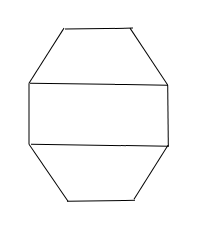# Draw a rough sketch of a regular octagon. (Use squared paper if you wish). Draw a rectangle by joining exactly four of the vertices of the octagon.

To do:

We have to draw a rough sketch of a regular octagon and draw a rectangle by joining exactly four of the vertices of the octagon.

Solution:We get a rectangle by joining exactly four of the vertices of the octagon as shown in the figure.